# SBI Clerk Mains Quantitative Aptitude Quiz- 15

## SBI Clerk Mains Quantitative Aptitude Quiz

Quantitative Aptitude is the most significant part of almost all competitive exams. Many candidates find difficulty in solving Quantitative Aptitude Questions. The only way to ace this section is to practice as many questions as possible. And, to help you practice more and more questions, we have come up with the SBI Clerk Mains Quantitative Aptitude Quiz. This SBI Clerk Mains Quantitative Aptitude Quiz is completely Free. This SBI Clerk Mains Quantitative Aptitude Quiz includes a variety of questions that are usually asked in mains exams. Candidates will be provided with a detailed solution for each question in this SBI Clerk Mains Quantitative Aptitude Quiz. This SBI Clerk Mains Quantitative Aptitude Quiz will assist candidates in better preparing for upcoming exams.

Directions (1-5): What approximate value will come in place of (x) in the following questions?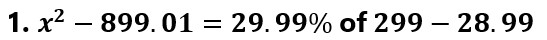(a) 25

(b) 40

(c) 38

(d) 35

(e) 31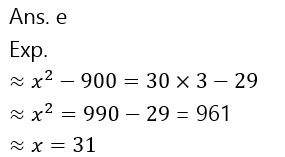(a) 200

(b) 350

(c) 400

(d) 300

(e) 450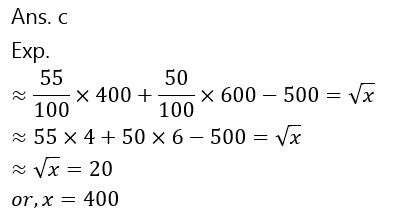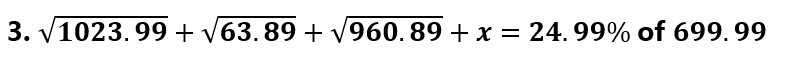(a) 104

(b) 111

(c) 96

(d) 90

(e) 120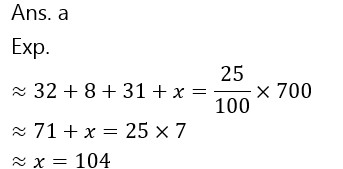(a) 10

(b) 8

(c) 17

(d) 13

(e) 19(a) 196

(b) 175

(c) 200

(d) 210

(e) 225Directions (6-10): The following questions are accompanied by three statements A, B and C. You have to determine which statement(s) is/are necessary/sufficient to answer the question.

1. What is the common difference of an arithmetic progression?

(A) Sixth term of the AP is 21 more than the third term of the AP.

(B) The first term of the AP is 8.

(C) The sum of third and seventh terms is 72.

(a) Only A alone

(b) Only C alone

(c) Only B and C together

(d) Either A alone or B and C together

(e) Only B alone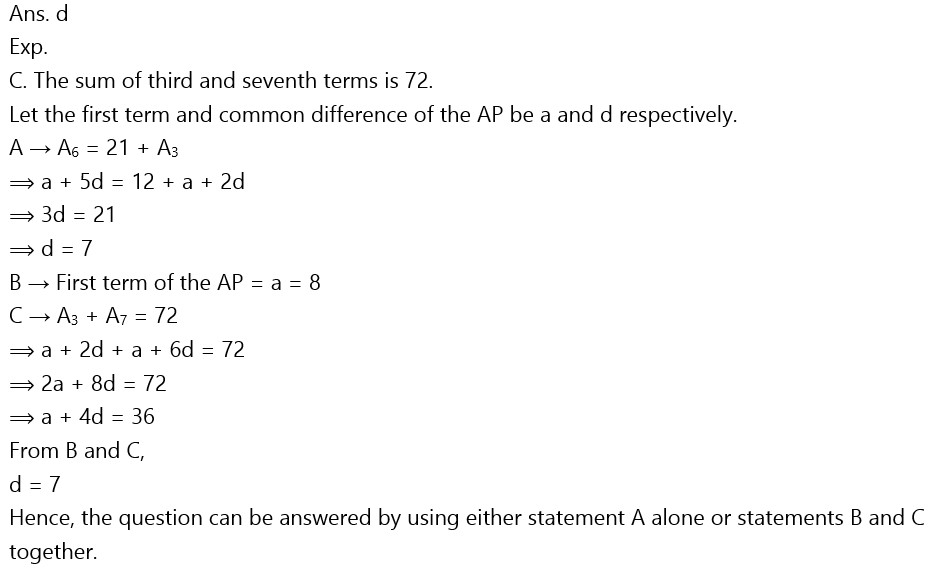1. What is the speed of the stream?

(A) The boat goes 49 km downstream in 3.5 hours.

(B) The ratio of the speed of boat in still water and the speed of stream is 6 : 1.

(C) The boat goes 10 km more in downstream than in upstream in 2.5 hours.

(a) Either C alone or A and B together

(b) Only A and B together

(c) Only A alone

(d) Only B alone

(e) Only C alone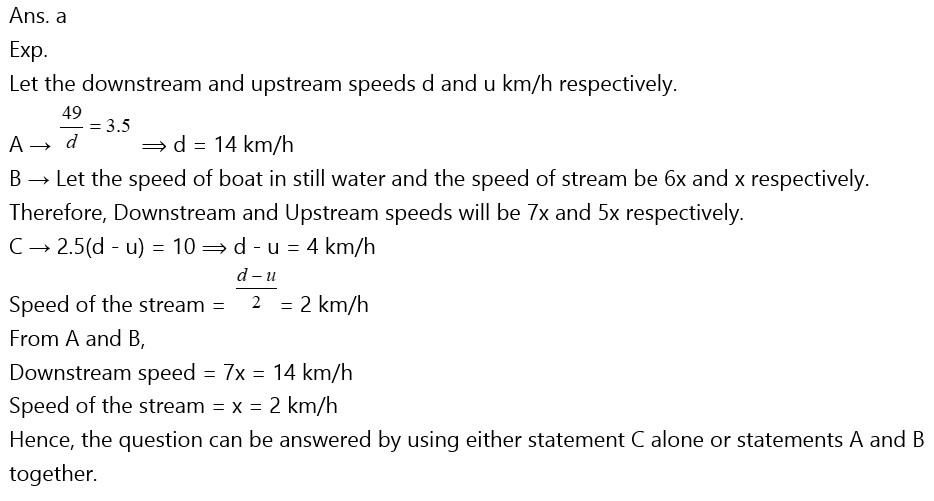1. What is the value of a two digit number?

(A) The ratio of the value of the number and the sum of the digits of that number is 4 : 1.

(B) The sum of the original number and the number obtained after interchanging the digits is 66.

(C) The number obtained after interchanging the digits is 18 more than the number itself.

(a) Only A and B together

(b) Only B and C together

(c) Only A and C together

(d) All the three together

(e) Any two of the three together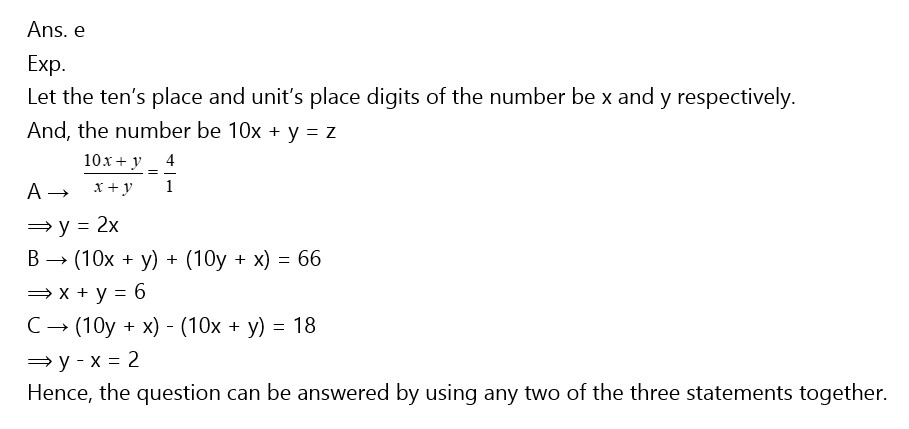1. Find out the length of a platform.

(A) A Train moving with a speed of 72 km/h crosses the platform in 50 sec.

(B) Ratio of the lengths of the train and the platform is 2 : 3.

(C) The length of the train is 400 meters.

(a) Any two of the three together

(b) All the three together are not sufficient

(c) Only A and B together

(d) Only B and C together

(e) Only A and C together1. What is the ratio of the efficiency of a man and a women?

(A) 10 men are as efficient as 12 women.

(B) 15 women can complete half of the work done by 25 men in same time.

(C) A man is 20% more efficient than a woman.

(a) Only A alone

(b) Either A alone or C alone

(c) Any one of the three alone

(d) Only C alone

(e) Either A alone or B alone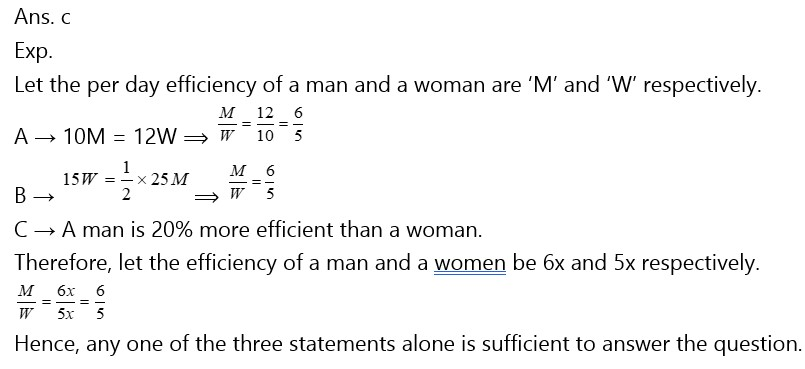### 2022 Preparation Kit PDF

#### Most important PDF’s for Bank, SSC, Railway and Other Government Exam : Download PDF Now

AATMA-NIRBHAR Series- Static GK/Awareness Practice Ebook PDF Get PDF here
The Banking Awareness 500 MCQs E-book| Bilingual (Hindi + English) Get PDF here
AATMA-NIRBHAR Series- Banking Awareness Practice Ebook PDF Get PDF here
Computer Awareness Capsule 2.O Get PDF here
AATMA-NIRBHAR Series Quantitative Aptitude Topic-Wise PDF 2020 Get PDF here
Memory Based Puzzle E-book | 2016-19 Exams Covered Get PDF here
Caselet Data Interpretation 200 Questions Get PDF here
Puzzle & Seating Arrangement E-Book for BANK PO MAINS (Vol-1) Get PDF here
ARITHMETIC DATA INTERPRETATION 2.O E-book Get PDF here
3